# Gravitational Red Shift

According to the principle of equivalence from general relativity, any frequency shift which can be shown to arise from acceleration of a radiating source could also be produced by the appropriate gravitational field. If a photon of frequency υ0 is emitted radially outward from the surface of a gravitational mass M, then the photon energy observed at a distance from the mass will be observed to be lower, or "red shifted". If observed at a great distance, we could denote the observed frequency as υ. The result of general relativity using the Schwarzschild metric is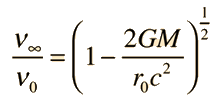which is valid for even strong gravitational fields. For weak gravitational fields or small displacements in a gravity field, one can make use of the approximation: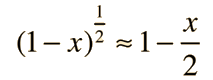to express the frequency shift between two locations as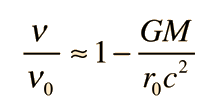Where υ0 represents a photon emitted closer to the gravitational mass. This form made practical some laboratory experiments using nuclear sources with the gravitational term expressed in terms of the gravitational acceleration g on the Earth's surface.

For the Harvard Tower experiment, the shifts in radiation frequency in the gravitational field were related to the relativistic doppler shift experienced from an accelerating light source.### Gravity and the photon

Applications:
 Harvard tower experiment
Index

General relativity ideas

References:
Carroll & Ostlie
Sec 17.1

 HyperPhysics***** Relativity R Nave
Go Back

# Harvard Tower ExperimentIn just 22.6 meters, the fractional gravitational red shift given by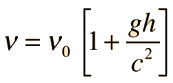is just 4.92 x 10-15 , but the Mossbauer effect with the 14.4 keV gamma ray from iron-57 has a high enough resolution to detect that difference. In the early 60's physicists Pound, Rebka,and Snyder at the Jefferson Physical Laboratory at Harvard measured the shift to within 1% of the predicted shift.

By just using the expression for gravitational potential energy near the Earth, and using the m in the relativistic energy expression, the gain in energy for a photon which falls distance h is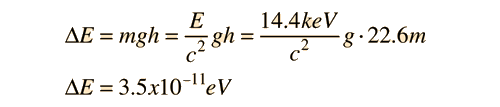Comparing the energy shifts on the upward and downward paths gives a predicted difference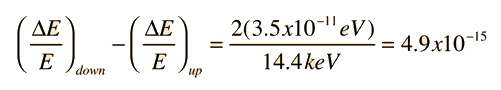The measured difference was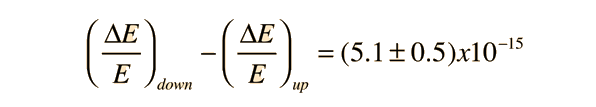The success of this experiment owed much to the care of Pound and Rebka in preparing the source. They electroplated cobalt-57 onto the surface of a thin sheet of iron and then heated the combination at 1220 K for an hour. The heat treatment caused the cobalt to diffuse into the iron to a depth of about 300 nm or 1000 atomic spacings. The source was then mounted on the cone of a loudspeaker driven at 10Hz to sweep the source velocity in a sinusoidal variation. The detector was a thin sheet of iron about 14 micrometers thick which was also annealed. The heat treatments were found to be crucial in obtaining high resolution.

Index

General relativity ideas

References: Harvard Tower

 HyperPhysics***** Relativity R Nave
Go Back

# Scout Rocket Experiment

In 1976 the Smithsonian Astrophysical Observatory sent aloft a Scout rocket to a height of 10,000 km. At this height, a clock should run 4.5 parts in 1010 faster than one on the Earth. During two hours of free fall from its maximum height, the rocket transmitted timing pulses from a maser oscillator which acted as a clock and which was compared with a similar clock on the ground. This result confirmed the gravitational time dilation relationship to within 0.01%.

### Gravity Probe A

Index

General relativity ideas

 HyperPhysics***** Relativity R Nave
Go Back

# Gravitational Time Dilation

A clock in a gravitational field runs more slowly according to the gravitational time dilation relationship from general relativity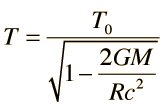This is distinct from the time dilation from relative motion
where T is the time interval measured by a clock far away from the mass. For a clock on the surface of the Earth, this expression becomesThis time dilation is about 1 part in 109. .....Show

Applications:
 Commercial airline experiment Scout rocket experiment
Index

General relativity ideas

 HyperPhysics***** Relativity R Nave
Go Back

# Time Dilation on Earth

The gravitational time dilation expression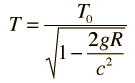has such a small second term in the denominator that excessive numerical accuracy is required to evaluate it directly. Using a binomial expansion :so that the first approximation to the time expression isThe numerical values were calculated using g = 9.8 m/s2, R = 6.38 x 106m (mean radius), and c= 3 x 108m/s.

Index

General relativity ideas

 HyperPhysics***** Relativity R Nave
Go Back